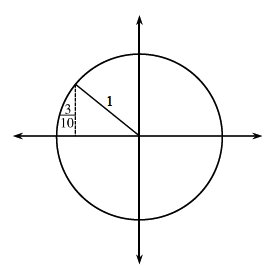### Home > CCA2 > Chapter 7 > Lesson 7.1.6 > Problem7-96

7-96.

Angle A is an obtuse angle with a sine of $\frac { 3 } { 10 }$. What is the tangent of angle A?

Draw this angle in a unit circle. In which quadrant(s) is sine positive?

Find the missing side of the triangle with the Pythagorean Theorem.
Remember that in a unit circle some sides are negative.

Tangent is $\frac{\text{opposite side}}{\text{adjacent side}}$.